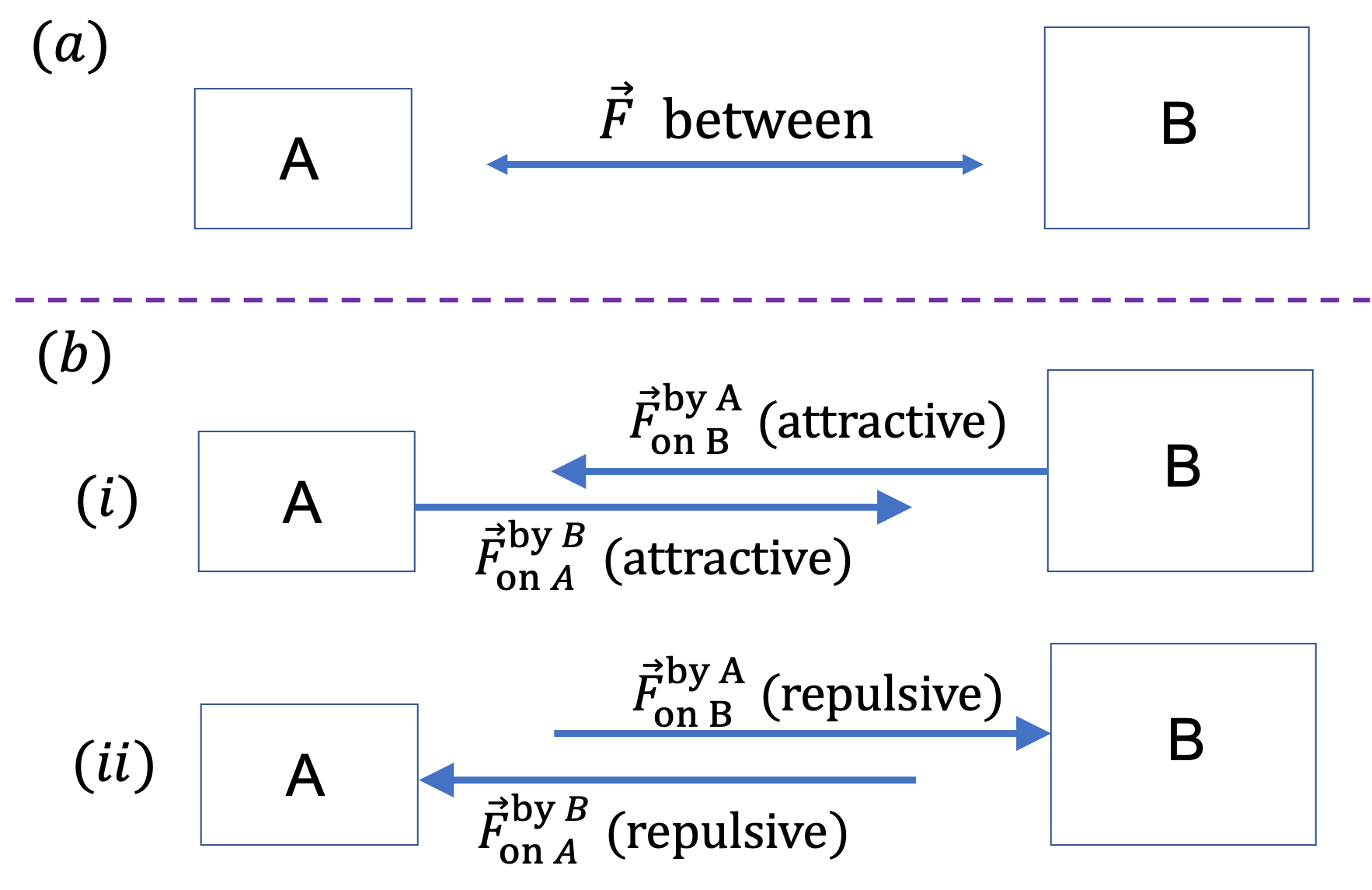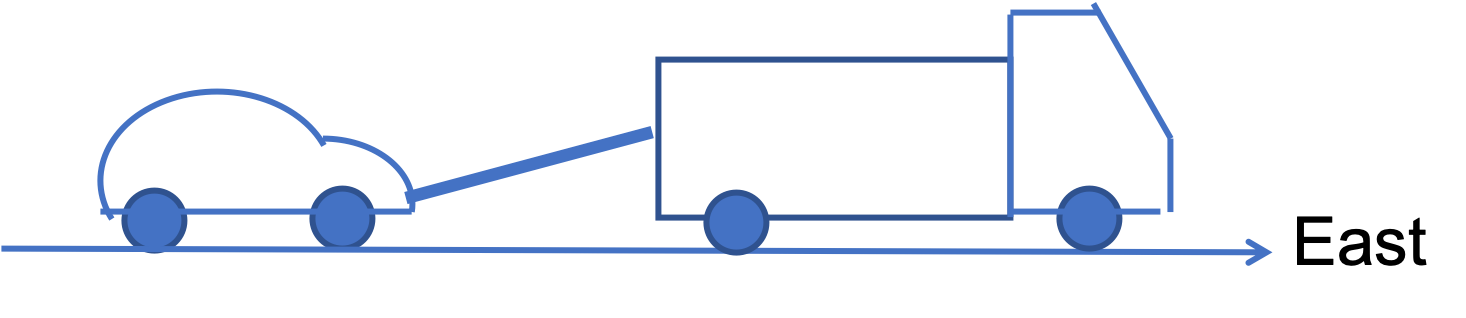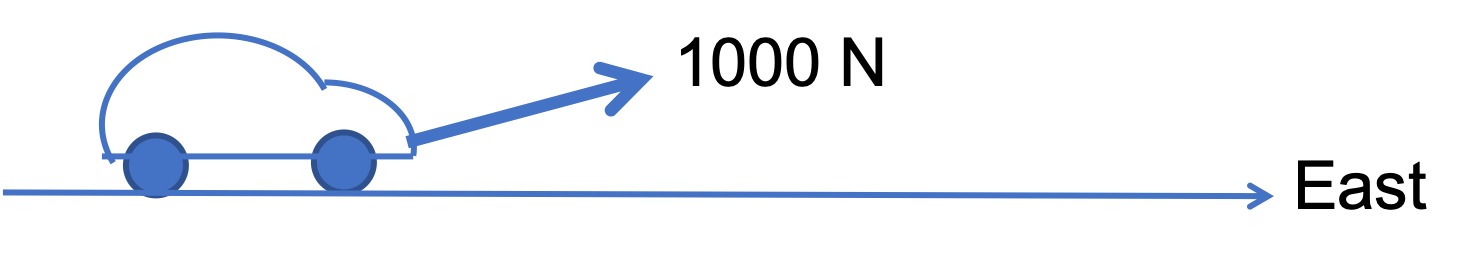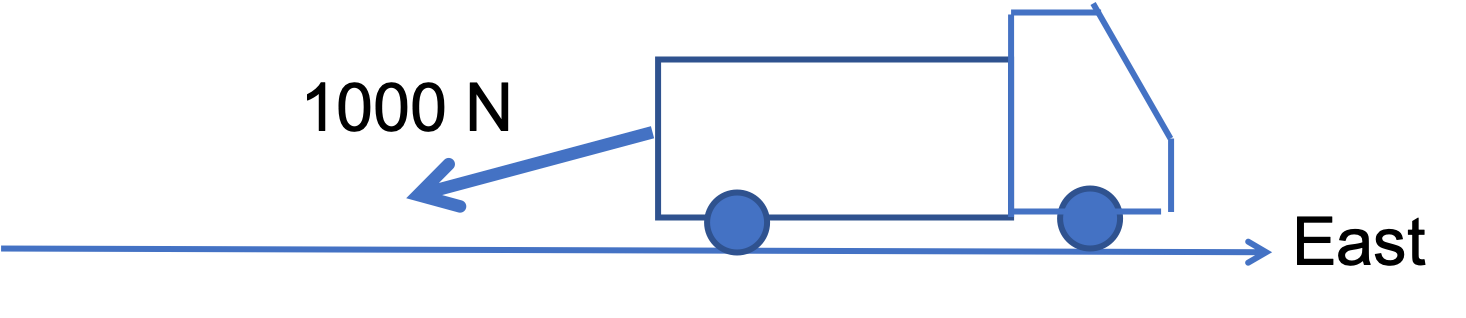## Section6.3Third Law of Motion

Newton's third of motion addresses the very nature of every force. Let $\vec F$ be the force between two bodies A and B. A confusing part of the concept of forces is that there are two different ways of speaking about them.

We can speak of a force between A and B or we can say that there are two separate forces, a force on A by B and another force on B by A, as illustrated in Figure 6.3.1. While “force between A and B” is a short way of talking about forces, actual forces are force on A by B and force on B by A; that is, there are actually two separate but related forces between any two objects.Figure 6.3.1. Two different ways of talking about forces: (a) The picture of “force between two bodies” is a conceptual way of speaking about forces, and (b) Separate forces on the two bodies emphasizes the agent (the by) and the target (the on) of the force. Only forces on A affect the motion of A.

Figure on the side panel illustrates the force pair that develops when you push on a wall. With your push, two forces are simultaneously generated, one acts on the wall and the other acts on you. They have the same magnitude but act in opposite directions and on different bodies.

Newton's third law is about the relation between the froce pairs, the force on A by B and force on B by A. The law says that their magnitudes are equal but their directions are exactly opposite. That is, as vectors, one force vector is equal to the negative of the other force vector.

\begin{equation} \vec F_{\text{on A}}^{\text{by B}} = - \vec F_{\text{on B}}^{\text{by A}}.\label{eq-third-law-forces}\tag{6.3.1} \end{equation}

How will we use this law in practice? We will see below that, according to Newton's second law of motion, the motion of A is affected only by the forces on A. But, sometimes, it is easier to figure out force by A rather than force on A. So, we use the third law to get force on A by first figuring out force by A and then reversing the direction.

A tow truck pulls a car with a force of $1000\text{ N}$ at a an angle of $15^{\circ}$ above horizon which is in the East direction.(a) Draw a picture of the force on the car by the truck, and describe the magnitude and direction of the force?

(b) Draw a picture of the force on the truck by the car, and describe the magnitude and direction of the force?

(c) If you use a Cartesian coordinate system with the positive $x$ axis towards East and the positive $y$ axis pointed up, what are the components of the two forces in (a) and (b)?

Hint

(a) and (b) Use "force on/by", (c) work out the force on the car.

(a) see solution, (b) see solution, (c) $\vec F_{\text{on car}} = (966\text{ N}, 259\text{ N})\text{,}$ and $\vec F_{\text{on truck}} = (-966\text{ N}, -259\text{ N})$

Solution 1 (a)

(a) The magnitude of the force is $1000 \text{ N}$ and the direction is $15^{\circ}$ above the East direction.Solution 2 (b)

(b) The magnitude of the force is $1000 \text{ N}$ and the direction is $15^{\circ}$ below the West direction.Solution 3 (c)

(c) Lets work out the components of forces on the car in (a), from which we just have to place negative signs on them to get those for force on the truck in (b), since they are in opposite directions.

\begin{align*} \amp F_x^{\text{on car}} = 1000\,\cos\,15^{\circ}=966\text{ N},\\ \amp F_y^{\text{on car}} = 1000\,\sin\,15^{\circ}=259\text{ N}. \end{align*}

Therefore, components of $\vec{F}^{\text{on truck}}$ will be negative of these values.

\begin{align*} \amp F_x^{\text{on truck}} = - 966\text{ N},\\ \amp F_y^{\text{on car}} = -259\text{ N}. \end{align*}